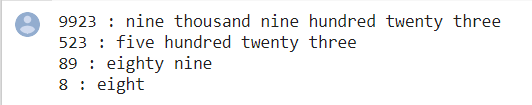top of page

# Number To Word Converter

Following is the implementation for the same. The code supports numbers up to 4 digits, i.e., numbers from 0 to 9999. Idea is to create arrays that store individual parts of output strings. One array is used for single digits, one for numbers from 10 to 19, one for 20, 30, 40, 50, .. etc, and one for powers of 10.

Here, I created the words list such as single_digit, two_digit, tens_multiple, and tens_power. so then I get the input from the user. this number divide by 10 by 10. then I mapped the word to each value of module of 10 values.

# Author: Aruna Nuwantha # Data Scientist program # NumToWord convertor # A function that prints # given number in words def convert_to_words(num): # Get number of digits # in given number l = len(num) # Base cases if (l == 0): print("empty string") return if (l > 4): print("Length more than 4 is not supported") return # The first string is not used, # it is to make array indexing simple single_digits = ["zero", "one", "two", "three", "four", "five", "six", "seven", "eight", "nine"] # The first string is not used, # it is to make array indexing simple two_digits = ["", "ten", "eleven", "twelve", "thirteen", "fourteen", "fifteen", "sixteen", "seventeen", "eighteen", "nineteen"] # The first two string are not used, # they are to make array indexing simple tens_multiple = ["", "", "twenty", "thirty", "forty", "fifty", "sixty", "seventy", "eighty", "ninety"] tens_power = ["hundred", "thousand"] # Used for debugging purpose only print(num, ":", end=" ") # For single digit number if (l == 1): print(single_digits[ord(num) - 48]) return # Iterate while num is not '\0' x = 0 while (x < len(num)): # Code path for first 2 digits if (l >= 3): if (ord(num[x]) - 48 != 0): print(single_digits[ord(num[x]) - 48], end=" ") print(tens_power[l - 3], end=" ") # here len can be 3 or 4 l -= 1 # Code path for last 2 digits else: # Need to explicitly handle # 10-19. Sum of the two digits # is used as index of "two_digits" # array of strings if (ord(num[x]) - 48 == 1): sum = (ord(num[x]) - 48 + ord(num[x+1]) - 48) print(two_digits[sum]) return # Need to explicitely handle 20 elif (ord(num[x]) - 48 == 2 and ord(num[x + 1]) - 48 == 0): print("twenty") return # Rest of the two digit # numbers i.e., 21 to 99 else: i = ord(num[x]) - 48 if(i > 0): print(tens_multiple[i], end=" ") else: print("", end="") x += 1 if(ord(num[x]) - 48 != 0): print(single_digits[ord(num[x]) - 48]) x += 1 if __name__ == '__main__': # Driver Code convert_to_words("9999") # Four Digits convert_to_words("500") # Three Digits convert_to_words("89") # Two Digits convert_to_words("9") # One Digits

#here is some test cases